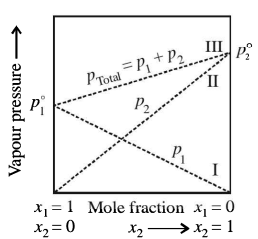# A plot of p1 or p2 vs the mole fractions x1 and x2  is given asIn this figure, lines I and II pass through the point for which.

1. A

${\mathrm{x}}_{1}\ne 1;{\mathrm{x}}_{2}=1$

2. B

${\mathrm{x}}_{1}={\mathrm{x}}_{2}\ne 1$

3. C

${\mathrm{x}}_{1}=1;{\mathrm{x}}_{2}\ne 1$

4. D

${\mathrm{x}}_{1}={\mathrm{x}}_{2}=1$

FREE Lve Classes, PDFs, Solved Questions, PYQ's, Mock Tests, Practice Tests, and Test Series!

+91

Verify OTP Code (required)

I agree to the terms and conditions and privacy policy.

### Solution:

lines I and II pass through the point for which, x1=x2=1

## Related contentJoin Infinity Learn Regular Class Program!

Sign up & Get instant access to FREE PDF's, solved questions, Previous Year Papers, Quizzes and Puzzles!

+91

Verify OTP Code (required)

I agree to the terms and conditions and privacy policy.